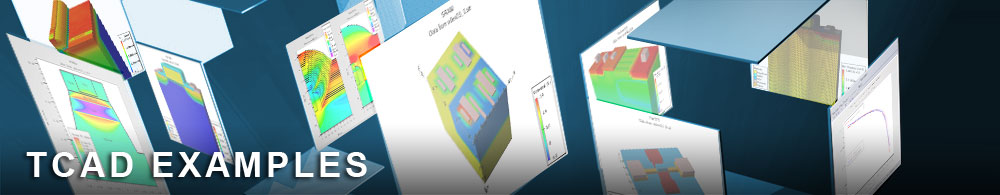Simple Gain Model

## laserex01.in : Simple Gain Model

Requires: Blaze/Laser
Minimum Versions: Atlas 5.28.1.R

This example demonstrates simulation of a buried heterostructure InP/InGaAsP laser diode using the simple gain model. It shows:

• Laser structure definition using Atlas syntax
• Material parameters specification
• Setting conventional physical models
• Device initial biasing
• Activating LASER module of Atlas
• Mesh definition for solving the Helmholtz wave equation
• Definition of laser physical models and their parameters including optical gain model
• Calculation of laser electrical and optical characteristics

At the beginning of the input file, the laser diode is described using Atlas structural syntax including mesh, regions, electrodes and doping distributions the same way as it is done for any conventional applications. The mesh statement uses the diag.flip parameter to make the mesh symmetrical with respect to the vertical center cross section. The mesh symmetry reflects the respective symmetry of the structure and may be important for calculating correctly the light intensity, local optical gain, and other internal physical distributions. Mesh spacings are specified to resolve heterojunctions in x and y directions.

The laser structure is composed of 10 regions. The active lasing region of the device is made of quaternary InGaAsP compound and surrounded by InP regions of n- and p- type. All heterojunctions are modeled as abrupt. The cathode electrode is defined in the center of the top of the device. The anode electrode extends across the device at the bottom. The composition parameters of the active region x.comp and y.comp are defined in the respective region statement. The composition parameters are used to calculate fundamental properties of the InGaAsP material. e.g. energy band gap, dielectric constant, densities of states in the conduction and valence bands, etc. using the default dependencies. The default values of the above parameters are used also for the InP regions.

Some other material parameters are specified in the material statements separately for InP and InGaAsP materials. These include electron and hole lifetimes (capture times) for the Shockley-Read-Hall recombination model, optical recombination constants, and low field mobilities. In addition the electron affinity for InGaAsP is explicitly defined to ensure correct energy band alignment. The parameter gain1min describing local optical gain and different from the default value is also given in the material statement for InGaAsP.

In two models statements a standard set of the Atlas/Blaze models is specified. In order to save computational time no LASER specific models are defined at this point. The LASER models are specified later in the input file after the initial bias has been increased. According to theory no laser emission will occur until the difference of quasi-Fermi levels in the active layer exceeds the material band gap. Therefore it is recommended to ramp the bias up to some value not greater than the band gap of the active region, and then enable the LASER models. In this example after obtaining the initial solution the anode voltage is ramped up to 0.6 V in the two-carrier solution mode.

The next part of the input file enables LASER specific models. It starts from defining a mesh for solution of the Helmholtz wave equation. The Helmholtz equation is solved on a uniform rectangular mesh that is independent of the triangular mesh used for electrical equations. The rectangular mesh and the region in which the Helmholtz equation is solved is defined using the lx.mesh and ly.mesh statements. The defined region must lie completely inside the BLAZE simulation domain and cover completely the active lasing region. The conventional models and LASER specific models are specified in the models statement. The LASER simulation is enabled by the parameter laser in this statement. In this example the the simple empirical gain model is activated by the parameter gainmod=2 . Here the optical gain is a function of electron and hole concentrations given in the form of analytical expression with empirical coefficients. The parameter las_maxch specifies the maximum allowable relative change in the photon densities between iterations. If not limited the rapid change in the photon density may cause convergence problems. The parameter photon_energy is used to specify the energy of the photons. If gainmod=2 is used it specifies only an initial estimate of the photon energy. Instead of photon_energy the parameter las_omega can be used to define the lasing frequency. The parameter cavity_length specifies the cavity length in the 3rd z-direction. For other LASER models and their parameters the default dependencies and values are applied.

In the LASER mode the anode voltage is ramped up to 1.7V. The I-V, light power, and total optical gain data, is saved in the log file. The final physical distributions in the structure are saved in the output structure file. The following results are visualized in TonyPlot:

• Conventional I-V characteristic
• Light power versus current
• Optical gain versus current
• Light intensity distribution over the structure

The behavior of the optical gain for the fundamental transverse mode illustrates its increase until the current reaches the threshold value and then it remains flat equal to the light losses (in this case 30cm-1).

To load and run this example, select the Load button in DeckBuild > Examples. This will copy the input file and any support files to your current working directory. Select the Run button in DeckBuild to execute the example.